RS Aggarwal Solutions: Mean, Median, Mode of Grouped Data, Cumulative Frequency Graph and Ogive- 3

# RS Aggarwal Solutions: Mean, Median, Mode of Grouped Data, Cumulative Frequency Graph and Ogive- 3 - RS Aggarwal Solutions for Class 10 Mathematics

## Multiple Choice Questions (MCQ)

Question for RS Aggarwal Solutions: Mean, Median, Mode of Grouped Data, Cumulative Frequency Graph and Ogive- 3
Try yourself:Which of the following is not a measure of central tendency?

Question for RS Aggarwal Solutions: Mean, Median, Mode of Grouped Data, Cumulative Frequency Graph and Ogive- 3
Try yourself:Which of the following cannot be determined graphically?

Question for RS Aggarwal Solutions: Mean, Median, Mode of Grouped Data, Cumulative Frequency Graph and Ogive- 3
Try yourself:Which of the following measures of central tendency is influenced by extreme values?

Question for RS Aggarwal Solutions: Mean, Median, Mode of Grouped Data, Cumulative Frequency Graph and Ogive- 3
Try yourself:The mode of a frequency distribution is obtained graphically from

Question for RS Aggarwal Solutions: Mean, Median, Mode of Grouped Data, Cumulative Frequency Graph and Ogive- 3
Try yourself:The median of a frequency distribution is found graphically with the help of

Question for RS Aggarwal Solutions: Mean, Median, Mode of Grouped Data, Cumulative Frequency Graph and Ogive- 3
Try yourself:The cumulative frequency table is useful in determining the

Question for RS Aggarwal Solutions: Mean, Median, Mode of Grouped Data, Cumulative Frequency Graph and Ogive- 3
Try yourself:The abscissa of the point of intersection of the Less Than Type and of the More Than Type cumulative frequency curves of a grouped data gives its

Question for RS Aggarwal Solutions: Mean, Median, Mode of Grouped Data, Cumulative Frequency Graph and Ogive- 3
Try yourself:If xi's are the midpoints of the class intervals of a grouped data, fi's are the corresponding frequencies and 7 is the mean then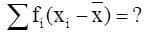Question for RS Aggarwal Solutions: Mean, Median, Mode of Grouped Data, Cumulative Frequency Graph and Ogive- 3
Try yourself:For finding the mean by using the formula,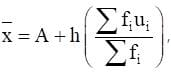we have ui = ?

Question for RS Aggarwal Solutions: Mean, Median, Mode of Grouped Data, Cumulative Frequency Graph and Ogive- 3
Try yourself:In the formula,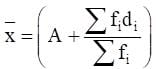for finding the mean of the grouped data, the di's are the deviations from A of

Question for RS Aggarwal Solutions: Mean, Median, Mode of Grouped Data, Cumulative Frequency Graph and Ogive- 3
Try yourself:While computing the mean of the grouped data, we Assume that the frequencies are

Question for RS Aggarwal Solutions: Mean, Median, Mode of Grouped Data, Cumulative Frequency Graph and Ogive- 3
Try yourself:The relation between mean, mode and median is

Question for RS Aggarwal Solutions: Mean, Median, Mode of Grouped Data, Cumulative Frequency Graph and Ogive- 3
Try yourself:If the 'less than type' ogive and 'more than type' ogive intersect each other at (20.5, 15.5) then the median of the given data is

Question for RS Aggarwal Solutions: Mean, Median, Mode of Grouped Data, Cumulative Frequency Graph and Ogive- 3
Try yourself:Consider the frequency distribution of the heights of 60 students of a class: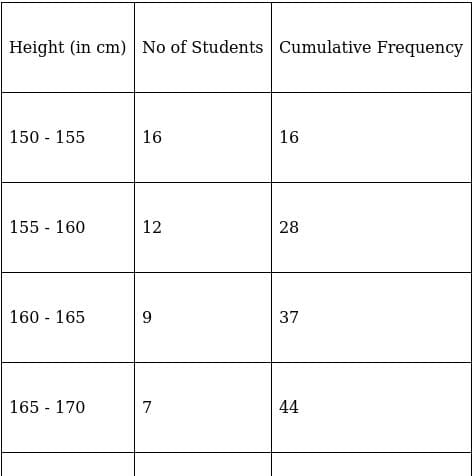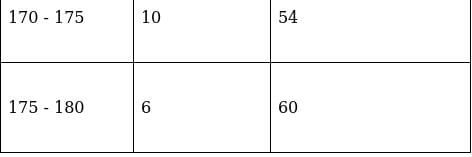The Sum of the lower limit of the modal class and the upper limit of the median class is

Question for RS Aggarwal Solutions: Mean, Median, Mode of Grouped Data, Cumulative Frequency Graph and Ogive- 3
Try yourself:Consider the following frequency distribution: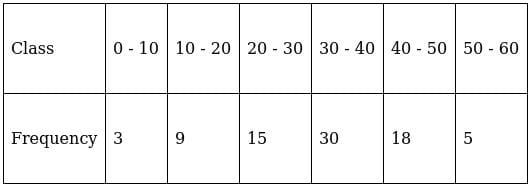The modal class is

Question for RS Aggarwal Solutions: Mean, Median, Mode of Grouped Data, Cumulative Frequency Graph and Ogive- 3
Try yourself:Mode = ?

Question for RS Aggarwal Solutions: Mean, Median, Mode of Grouped Data, Cumulative Frequency Graph and Ogive- 3
Try yourself:Median = ?

Question for RS Aggarwal Solutions: Mean, Median, Mode of Grouped Data, Cumulative Frequency Graph and Ogive- 3
Try yourself:If the mean and median of a set of numbers are 8.9 and 9 respectively then the mode will be

Question for RS Aggarwal Solutions: Mean, Median, Mode of Grouped Data, Cumulative Frequency Graph and Ogive- 3
Try yourself:Look at the frequency distribution table given below: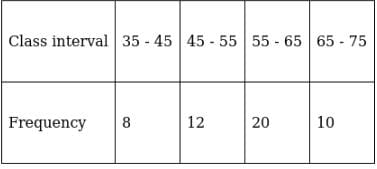The median of the above distribution is

Question for RS Aggarwal Solutions: Mean, Median, Mode of Grouped Data, Cumulative Frequency Graph and Ogive- 3
Try yourself:Consider the following table: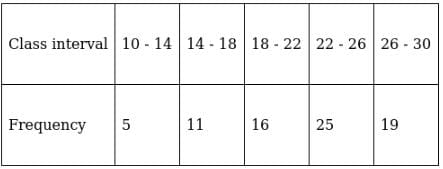The mode of the above data is

Question for RS Aggarwal Solutions: Mean, Median, Mode of Grouped Data, Cumulative Frequency Graph and Ogive- 3
Try yourself:The mean and mode of a frequency distribution are 28 and 16 respectively. The median is

Question for RS Aggarwal Solutions: Mean, Median, Mode of Grouped Data, Cumulative Frequency Graph and Ogive- 3
Try yourself:The median and mode of a frequency distribution are 26 and 29 respectively. Then, the mean is

Question for RS Aggarwal Solutions: Mean, Median, Mode of Grouped Data, Cumulative Frequency Graph and Ogive- 3
Try yourself:For a symmetrical frequency distribution, we have

Question for RS Aggarwal Solutions: Mean, Median, Mode of Grouped Data, Cumulative Frequency Graph and Ogive- 3
Try yourself:Look at the cumulative frequency distribution table given below: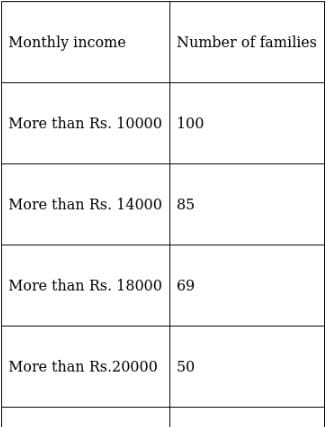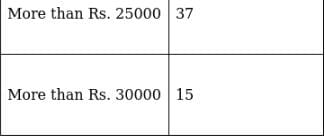Number of families having income range 20000 to 25000 is

Question for RS Aggarwal Solutions: Mean, Median, Mode of Grouped Data, Cumulative Frequency Graph and Ogive- 3
Try yourself:The median of first 8 prime numbers is

Question for RS Aggarwal Solutions: Mean, Median, Mode of Grouped Data, Cumulative Frequency Graph and Ogive- 3
Try yourself:The mean of 20 numbers is zero. Of them, at the most, how many may be greater than zero?

Question for RS Aggarwal Solutions: Mean, Median, Mode of Grouped Data, Cumulative Frequency Graph and Ogive- 3
Try yourself:If the median of the data 4,7,x - 1,X - 3,l6,25, written in ascending order, is 13 then x is equal to

Question for RS Aggarwal Solutions: Mean, Median, Mode of Grouped Data, Cumulative Frequency Graph and Ogive- 3
Try yourself:The mean of 2, 7, 6 and x is 15 and the mean of 18, 1, 6, x and y is 10. What is the value of y?

Question for RS Aggarwal Solutions: Mean, Median, Mode of Grouped Data, Cumulative Frequency Graph and Ogive- 3
Try yourself:Each question consists of two statements, namely, Assertion (A) and Reason (R). For selecting the correct answer, use the following code: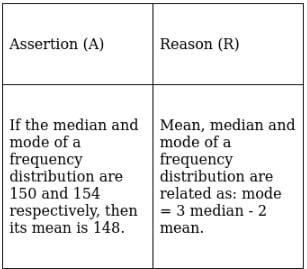Question for RS Aggarwal Solutions: Mean, Median, Mode of Grouped Data, Cumulative Frequency Graph and Ogive- 3
Try yourself:Each question consists of two statements, namely, Assertion (A) and Reason (R). For selecting the correct answer, use the following code: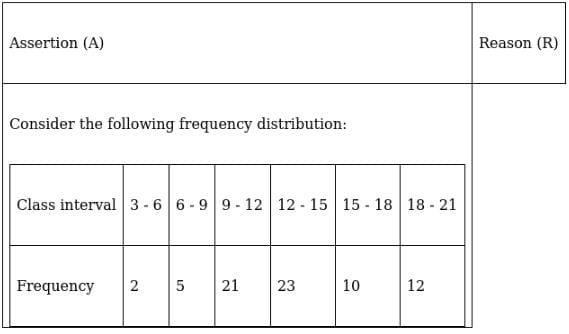The mode of the above data is 12.4.
The value of the variable which occurs most often is the mode.

The document RS Aggarwal Solutions: Mean, Median, Mode of Grouped Data, Cumulative Frequency Graph and Ogive- 3 | RS Aggarwal Solutions for Class 10 Mathematics is a part of the Class 10 Course RS Aggarwal Solutions for Class 10 Mathematics.
All you need of Class 10 at this link: Class 10

53 docs|15 tests

## RS Aggarwal Solutions for Class 10 Mathematics

53 docs|15 testsExplore Courses for Class 10 examSignup to see your scores go up within 7 days! Learn & Practice with 1000+ FREE Notes, Videos & Tests.
10M+ students study on EduRev
Track your progress, build streaks, highlight & save important lessons and more!
Related Searches

,

,

,

,

,

,

,

,

,

,

,

,

,

,

,

,

,

,

,

,

,

,

,

,

,

,

,

,

,

,

;